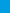# Ace the ALEKS Math in 30 Days The Ultimate Crash Course to Beat the ALEKS Math TestAce the ALEKS Math in 30 Days is a perfect prep book for students who want to learn ALEKS Math topics in a structured manner. This comprehensive book with other Effortless Math books have helped thousands of students succeed on the ALEKS Mathematics Placement Assessment Test.\$15.99\$9.99
-38% only \$9.99

Looking for a perfect prep book to help you prepare for the ALEKS Mathematics Placement Assessment Test? If yes, then you’ve come to the right place! Ace the ALEKS Math in 30 Days, which reflects the 2019 and 2020 test procedures and guidelines, is the only book you will ever need to fully prepare for the ALEKS Math test. This comprehensive preparation book covers all mathematics topics that you will be tested. This completely updated version of the book can help you use the best method and the right strategies to prepare for the ALEKS Mathematics Placement Assessment in a short period of time. All mathematics topics that you will need to learn are divided into 28 days and you will learn a few topics each day. You only need to spend about 2-3 hours a day to be well prepared for the test.

The book begins with basic mathematics topics such as fractions, decimals, mixed numbers, percentage, order of operations, ratios and proportions, ect. Then it gradually gets into algebra, geometry and more advanced topics such as Statistics and Probability, Matrices, Logarithms, Trigonometric Functions, etc.

For each topic, a step by step instruction to tackle each types of questions is provided. Many detailed examples and exercises in the book will help you understand the topic thoroughly.

The surest way to succeed on the Accuplacer test is by studying and reviewing the ALEKS Math sample questions and practice tests. That’s why there are two complete and realistic ALEKS Mathematics Placement Assessment Tests on days 29 and 30. All practice questions are followed by detailed answers to help you find your weak areas, improve your knowledge of mathematics, and ace the ALEKS Math test.

The winning formula and effective instruction method of the book have helped thousands of students to learn mathematics topics successfully, measure their knowledge of math, and succeed on the ALEKS Math test.

## Reviews

There are no reviews yet.

?